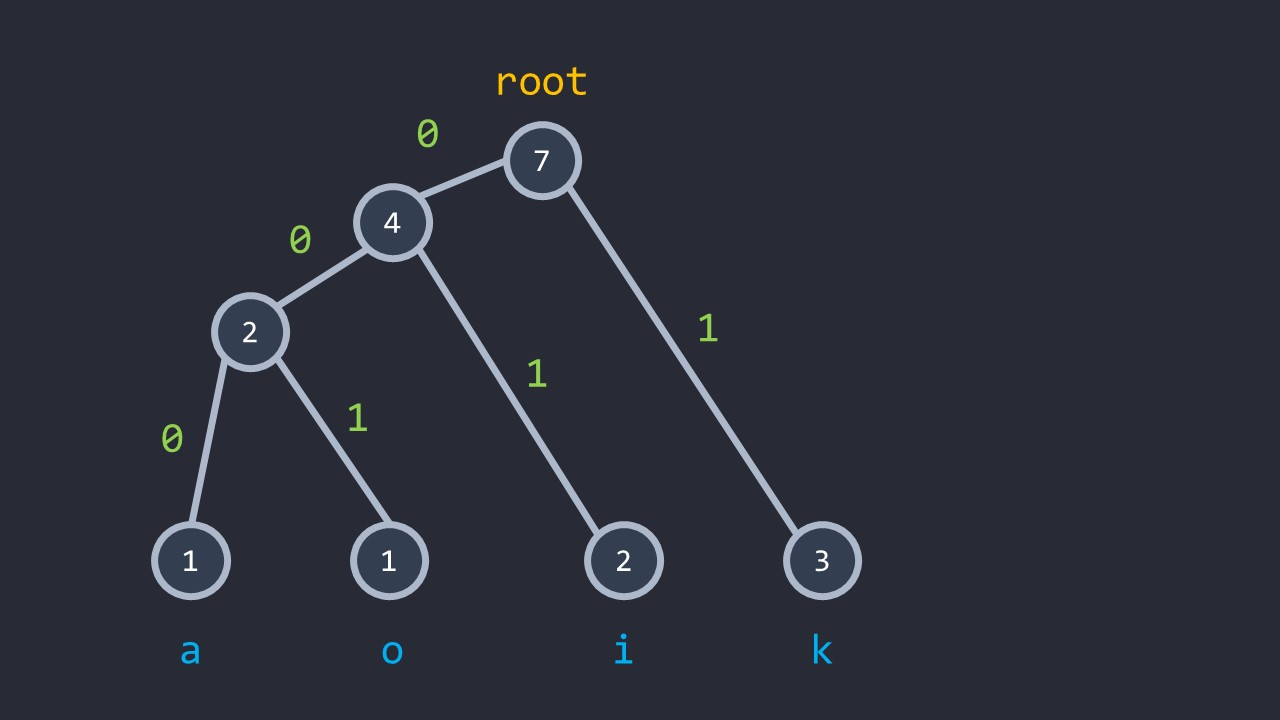11 篇文章 0 订阅

# 哈夫曼树&编码

https://blog.csdn.net/qq_29519041/article/details/81428934

## 讲解构造 \color{#000}\texttt{讲解构造}

### 怎么构造最优编码？code

#include <bits/stdc++.h>
using namespace std;

//&Start
#define lng long long
#define lit long double
const int inf=0x3f3f3f3f;
const lng Inf=1e17;

//&Main
const int N=510;
int n,w[N<<1],cnt,dep[N<<1],ans;
vector<int> g[N<<1];
class cmp{
public:
bool operator()(int x,int y){//通过比较wi得到小顶堆
return w[x]>w[y];
}
};
priority_queue<int,vector<int>,cmp> q;
void dfs(int x){//求li
for(auto to:g[x]) dep[to]=dep[x]+1,dfs(to);
}
int main(){
scanf("%d",&n);
for(int i=1;i<=n;i++)
scanf("%d",&w[++cnt]),q.push(cnt);
while(q.size()>1){
int x=q.top(),y;
q.pop();y=q.top();q.pop();
w[++cnt]=w[x]+w[y];
g[cnt].push_back(x);
g[cnt].push_back(y);
q.push(cnt);
}
dfs(cnt);
for(int i=1;i<=n;i++)
ans+=w[i]*dep[i];
printf("%d\n",ans);
return 0;
}


## 经典例题 \color{#000}\texttt{经典例题}

[NOIp2004]合并果子

### 荷马史诗

[NOI2015]荷马史诗

code

#include <bits/stdc++.h>
using namespace std;

//&Start
#define lng long long
#define lit long double
const int inf=0x3f3f3f3f;
const lng Inf=1e17;

//&Main
const int N=1e5+10;
int n,k,cnt;
lng w[N<<1],dep[N<<1],ans,mx;//不开long long见祖宗
vector<int> g[N<<1];
class cmp{
public:
bool operator()(int x,int y){
if(w[x]==w[y]) return x>y;//特判排序
return w[x]>w[y];
}
};
priority_queue<int,vector<int>,cmp> q;
void dfs(int x){
for(auto to:g[x]) dep[to]=dep[x]+1,dfs(to);
}
int main(){
scanf("%d%d",&n,&k);
for(int i=1;i<=n;i++)
scanf("%lld",&w[++cnt]),q.push(cnt);
while((q.size()-1)%(k-1)) q.push(0);//插0
while(q.size()>1){
++cnt;
for(int i=1;i<=k;i++){//k叉哈夫曼树
int x=q.top();q.pop();
g[cnt].push_back(x);
w[cnt]+=w[x];
}
q.push(cnt);
}
dfs(cnt);
for(int i=1;i<=n;i++)
ans+=w[i]*dep[i],mx=max(mx,dep[i]);
printf("%lld\n%lld\n",ans,mx);
return 0;
}


09-102万+02-049705
02-076596
09-172万+
06-176万+
04-193万+
11-292万+
04-303万+
11-04454
08-10248
04-25704
12-14392
10-291万+
09-086320
08-062079
10-164917
11-212万+点击重新获取扫码支付余额充值